# TypeError: 'float' object is not iterable in Python [Fixed]Last updated: Jan 31, 2023
6 min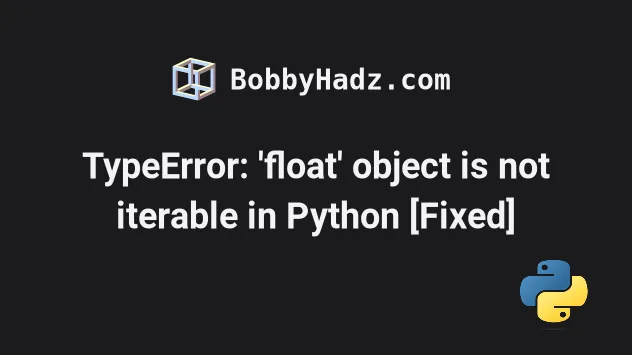## #TypeError: 'float' object is not iterable in Python

The Python "TypeError: 'float' object is not iterable" occurs when we try to iterate over a float or pass a float to a built-in function like `list()` or `tuple()`.

To solve the error, use the `range()` built-in function to iterate over a range.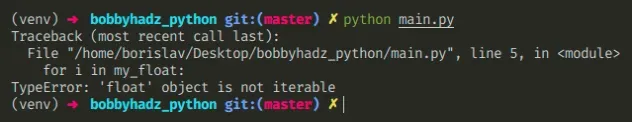Here is an example of how the error occurs.

main.py
```Copied!```my_float = 3.1

# ⛔️ TypeError: 'float' object is not iterable
for i in my_float:
print(i)
``````

We are trying to iterate over a floating-point number, but floats are not iterable.

## #Use the `range()` function to iterate over a range

We can use the `range()` built-in function to iterate over a range.

main.py
```Copied!```my_float = 3.1

for i in range(int(my_float)):
print(i)  # 👉️ 0 1 2
``````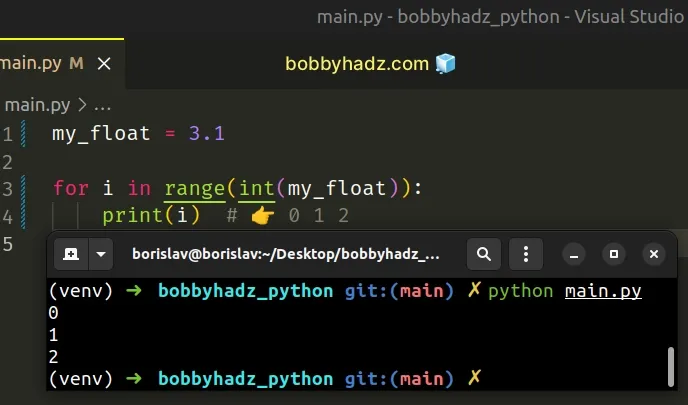Notice that we also used the `int()` function to convert the float to an integer because the range function expects an integer argument.

The range function is commonly used for looping a specific number of times in for loops and takes the following parameters:

NameDescription
`start`An integer representing the start of the range (defaults to `0`)
`stop`Go up to, but not including the provided integer
`step`Range will consist of every N numbers from `start` to `stop` (defaults to `1`)
If you only pass a single argument to the `range()` constructor, it is considered to be the value for the `stop` parameter.

If values for the `start` and `stop` parameters are provided, the `start` value is inclusive, whereas the `stop` value is exclusive.

main.py
```Copied!```my_float = 3.1

for i in range(1, int(my_float)):
print(i)  # 👉️ 1 2
``````

## #Using a `try/except` statement to handle the error

You can use a try/except statement to handle the `TypeError`.

main.py
```Copied!```my_float = 3.1

try:
for i in my_float:
print(i)
except TypeError:
# The object is not iterable
# <class 'float'>
print('The object is not iterable')
print(type(my_float))
``````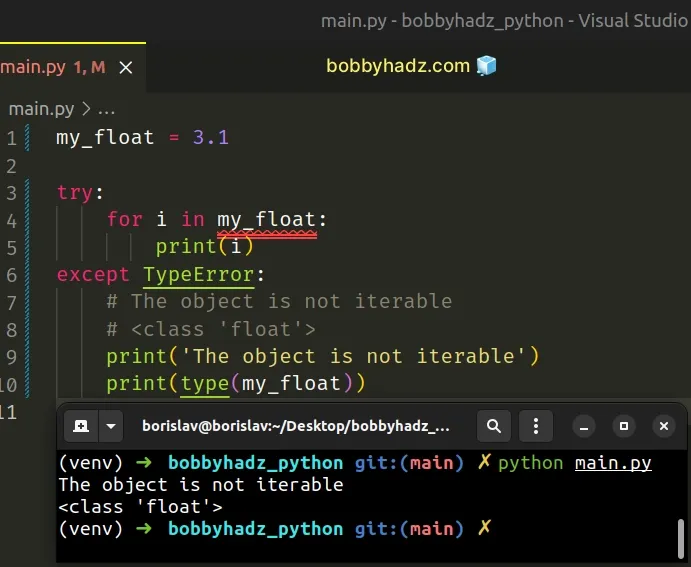The `try` statement tries to iterate over the value and if an exception is raised, the `except` block runs.

You can print the value and its type in the `except` block to debug your code.

## #Passing a float to a built-in constructor

Another common cause of the error is passing a float to the built-in constructors, e.g. `list()`, `dict()`, `tuple()` and set().

The following 4 calls to the built-in constructors cause the error.

main.py
```Copied!```my_float = 3.1

# ⛔️ TypeError: 'float' object is not iterable
list(my_float)

dict(my_float)

tuple(my_float)

set(my_float)
``````

To solve the error, we have to correct the assignment and figure out where the float value is coming from.

Here are working examples of using the 4 built-ins.

main.py
```Copied!```l = list(['a', 'b', 'c'])
print(l)  # 👉️ ['a', 'b', 'c']

print(d)  # 👉️ {'name': 'Bobby Hadz', 'age': 30}

t = tuple([1, 2, 3])
print(t)  # 👉️ (1, 2, 3)

s = set(['a', 'b', 'a'])
print(s)  # 👉️ {'a', 'b'}
``````

You have to figure out where the float value came from and correct the assignment.

You might also get the error when calling the built-in sum() function with a floating-point number.

main.py
```Copied!```# ⛔️ TypeError: 'float' object is not iterable
result = sum(3.1, 4.5)
``````

The `sum()` function takes an iterable as an argument, so we can wrap the values in a list.

main.py
```Copied!```result = sum([3.1, 4.5])

print(result) # 👉️ 7.6
``````

Passing the two floating-point numbers in a list resolves the issue because the function takes an iterable.

If you need to iterate with both the index and the current character or element, use the `enumerate()` function.

main.py
```Copied!```my_str = 'hello'

for idx, c in enumerate(my_str):
print(idx, c)  # 👉️ 0 h, 1 e, 2 l...

my_list = ['a', 'b', 'c']

for idx, el in enumerate(my_list):
print(idx, el)  # 👉️ 0 a, 1 b, 2 c
``````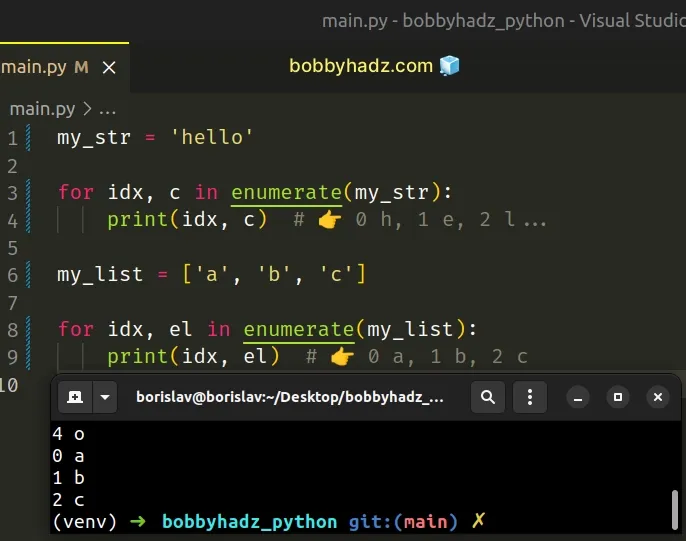## #Checking if an object is iterable

If you need to check if an object is iterable, use a `try/except` statement.

main.py
```Copied!```my_str = 'hello'

try:
my_iterator = iter(my_str)

for i in my_iterator:
print(i) # 👉️ h, e, l, l, o
except TypeError as te:
print(te)
``````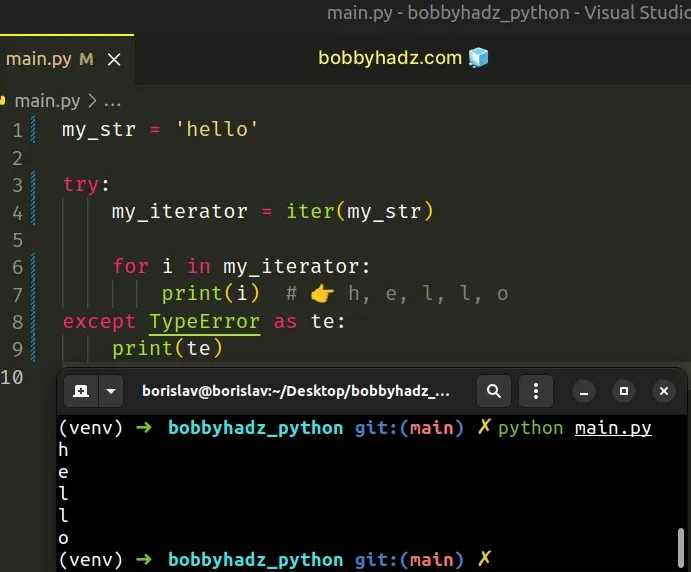The iter() function raises a `TypeError` if the passed-in value doesn't support the `__iter__()` method or the sequence protocol (the `__getitem__()` method).

If we pass a non-iterable object like a float to the `iter()` function, the `except` block is run.

main.py
```Copied!```my_float = 3.5

try:
my_iterator = iter(my_float)

for i in my_iterator:
print(i)
except TypeError as te:
print(te)  # 👉️ 'float' object is not iterable
``````

Examples of iterables include all sequence types (`list`, `str`, `tuple`) and some non-sequence types like `dict`, file objects and other objects that define an `__iter__()` or a `__getitem__()` method.

## #TypeError: argument of type 'float' is not iterable (Python)

The Python "TypeError: argument of type 'float' is not iterable" occurs when we use the membership test operators (in and not in) with a float value.

To solve the error, correct the assignment or convert the float to a string, e.g. `str(my_float)`.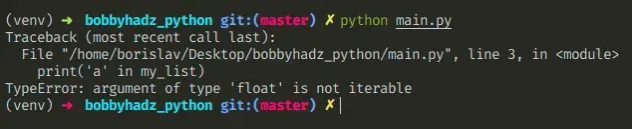Here is an example of how the error occurs.

main.py
```Copied!```my_list = 3.5

# ⛔️ TypeError: argument of type 'float' is not iterable
print('a' in my_list)
``````

We tried to use a membership test operator with a float value and got the error.

Chances are you meant to use the operator with an iterable, e.g. a string or a list.

## #Convert the float to a string

One way to solve the error is to convert the float to a string.

main.py
```Copied!```my_str = 3.5

print('a' in str(my_str))  # 👉️ False

print('3' in str(my_str))  # 👉️ True
``````

Strings are iterable, so converting the floating-point number to a string solves the issue.

## #Track down where the variable got assigned a float

However, the best way to solve the error is to track down where the variable got assigned a float and correct the assignment.

For example, you can reassign the variable if it stores a value of an unexpected type.

main.py
```Copied!```my_list = 3.5

if not isinstance(my_list, list):
my_list = []

print('a' in my_list)  # 👉️ False
``````

We check if the `my_list` variable doesn't store a list, and if it doesn't, we set it to an empty list before using the `in` operator.

You can use this approach with any other object, e.g. `str`, `dict`, `tuple`, etc.

main.py
```Copied!```my_str = 3.5

if not isinstance(my_str, str):
my_str = ""

print('a' in my_str)  # 👉️ False
``````

If the variable isn't a string, we set it to an empty string.

## #Checking if the value is not a float before using the `in` operator

Alternatively, you can check if the value is not a float before using the `in` or `not in` operators.

main.py
```Copied!```my_str = 3.5

if not isinstance(my_str, float):
print('a' in my_str)
else:
# 👇️ this runs
print('value is a float')
``````

We check if the value is not an instance of the `float` class, and if it isn't, we use the `in` operator to test for membership.

However, it's safer to check if the value is of the expected type, e.g. a `str`, a `list`, or a `dict`.

main.py
```Copied!```my_str = 3.5

if isinstance(my_str, str):
print('a' in my_str)
else:
# 👉️ this runs
print('value is not a string')
``````

Our `if` statement checks if the value is an instance of the `str` class and if it is, we use the `in` operator.

The in operator tests for membership. For example, `x in s` evaluates to `True` if `x` is a member of `s`, otherwise it evaluates to `False`.

main.py
```Copied!```my_str = 'bobbyhadz.com'

print('bobby' in my_str)  # 👉️ True

print('another' in my_str)  # 👉️ False
``````

`x` not in `s` returns the negation of `x` in `s`.

All built-in sequences and set types support the `in` and `not in` operators.

When used with a dictionary, the operators check for the existence of the specified key in the `dict` object.